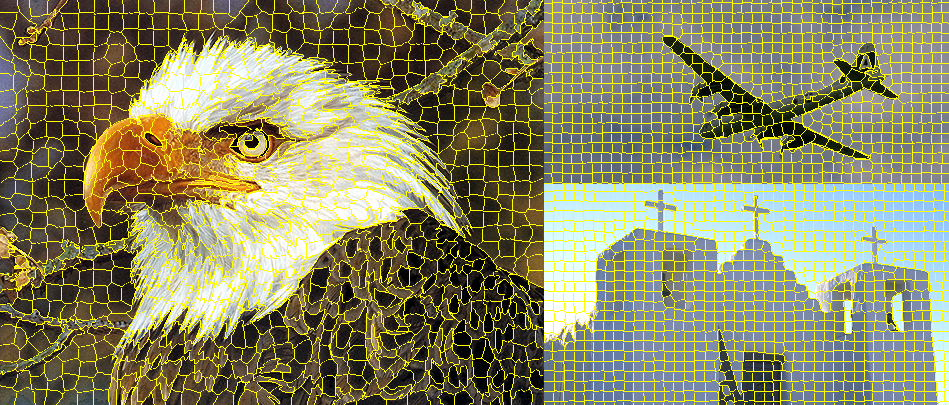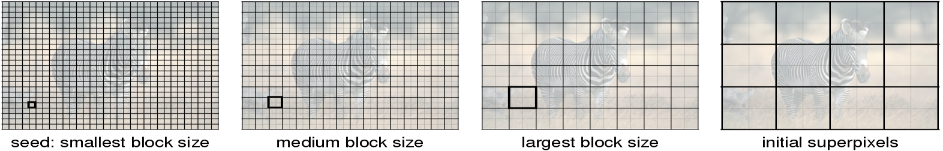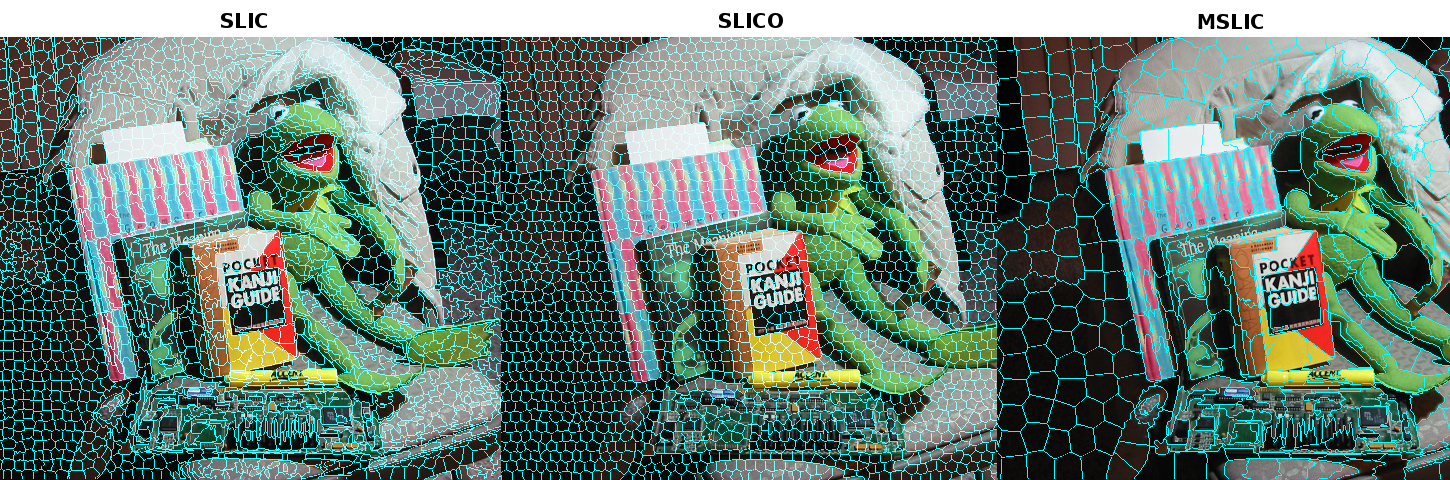OpenCV  4.0.0-beta Open Source Computer Vision

## Classes

class  cv::ximgproc::SuperpixelLSC
Class implementing the LSC (Linear Spectral Clustering) superpixels algorithm described in . More...

class  cv::ximgproc::SuperpixelSEEDS
Class implementing the SEEDS (Superpixels Extracted via Energy-Driven Sampling) superpixels algorithm described in  . More...

class  cv::ximgproc::SuperpixelSLIC
Class implementing the SLIC (Simple Linear Iterative Clustering) superpixels algorithm described in . More...

## Enumerations

enum  cv::ximgproc::SLICType {
cv::ximgproc::SLIC = 100,
cv::ximgproc::SLICO = 101,
cv::ximgproc::MSLIC = 102
}

## Functions

Ptr< SuperpixelLSCcv::ximgproc::createSuperpixelLSC (InputArray image, int region_size=10, float ratio=0.075f)
Class implementing the LSC (Linear Spectral Clustering) superpixels. More...

Ptr< SuperpixelSEEDScv::ximgproc::createSuperpixelSEEDS (int image_width, int image_height, int image_channels, int num_superpixels, int num_levels, int prior=2, int histogram_bins=5, bool double_step=false)
Initializes a SuperpixelSEEDS object. More...

Ptr< SuperpixelSLICcv::ximgproc::createSuperpixelSLIC (InputArray image, int algorithm=SLICO, int region_size=10, float ruler=10.0f)
Initialize a SuperpixelSLIC object. More...

## § SLICType

Enumerator
SLIC
Python: cv.ximgproc.SLIC
SLICO
Python: cv.ximgproc.SLICO
MSLIC
Python: cv.ximgproc.MSLIC

## § createSuperpixelLSC()

 Ptr cv::ximgproc::createSuperpixelLSC ( InputArray image, int region_size = 10, float ratio = 0.075f )
Python:
retval=cv.ximgproc.createSuperpixelLSC(image[, region_size[, ratio]])

Class implementing the LSC (Linear Spectral Clustering) superpixels.

Parameters
 image Image to segment region_size Chooses an average superpixel size measured in pixels ratio Chooses the enforcement of superpixel compactness factor of superpixel

The function initializes a SuperpixelLSC object for the input image. It sets the parameters of superpixel algorithm, which are: region_size and ruler. It preallocate some buffers for future computing iterations over the given image. An example of LSC is ilustrated in the following picture. For enanched results it is recommended for color images to preprocess image with little gaussian blur with a small 3 x 3 kernel and additional conversion into CieLAB color space.image

## § createSuperpixelSEEDS()

 Ptr cv::ximgproc::createSuperpixelSEEDS ( int image_width, int image_height, int image_channels, int num_superpixels, int num_levels, int prior = 2, int histogram_bins = 5, bool double_step = false )
Python:
retval=cv.ximgproc.createSuperpixelSEEDS(image_width, image_height, image_channels, num_superpixels, num_levels[, prior[, histogram_bins[, double_step]]])

Initializes a SuperpixelSEEDS object.

Parameters
 image_width Image width. image_height Image height. image_channels Number of channels of the image. num_superpixels Desired number of superpixels. Note that the actual number may be smaller due to restrictions (depending on the image size and num_levels). Use getNumberOfSuperpixels() to get the actual number. num_levels Number of block levels. The more levels, the more accurate is the segmentation, but needs more memory and CPU time. prior enable 3x3 shape smoothing term if >0. A larger value leads to smoother shapes. prior must be in the range [0, 5]. histogram_bins Number of histogram bins. double_step If true, iterate each block level twice for higher accuracy.

The function initializes a SuperpixelSEEDS object for the input image. It stores the parameters of the image: image_width, image_height and image_channels. It also sets the parameters of the SEEDS superpixel algorithm, which are: num_superpixels, num_levels, use_prior, histogram_bins and double_step.

The number of levels in num_levels defines the amount of block levels that the algorithm use in the optimization. The initialization is a grid, in which the superpixels are equally distributed through the width and the height of the image. The larger blocks correspond to the superpixel size, and the levels with smaller blocks are formed by dividing the larger blocks into 2 x 2 blocks of pixels, recursively until the smaller block level. An example of initialization of 4 block levels is illustrated in the following figure.image

## § createSuperpixelSLIC()

 Ptr cv::ximgproc::createSuperpixelSLIC ( InputArray image, int algorithm = SLICO, int region_size = 10, float ruler = 10.0f )
Python:
retval=cv.ximgproc.createSuperpixelSLIC(image[, algorithm[, region_size[, ruler]]])

Initialize a SuperpixelSLIC object.

Parameters
 image Image to segment algorithm Chooses the algorithm variant to use: SLIC segments image using a desired region_size, and in addition SLICO will optimize using adaptive compactness factor, while MSLIC will optimize using manifold methods resulting in more content-sensitive superpixels. region_size Chooses an average superpixel size measured in pixels ruler Chooses the enforcement of superpixel smoothness factor of superpixel

The function initializes a SuperpixelSLIC object for the input image. It sets the parameters of choosed superpixel algorithm, which are: region_size and ruler. It preallocate some buffers for future computing iterations over the given image. For enanched results it is recommended for color images to preprocess image with little gaussian blur using a small 3 x 3 kernel and additional conversion into CieLAB color space. An example of SLIC versus SLICO and MSLIC is ilustrated in the following picture.image